# Google Code Jam Archive — Qualification Round 2022 problems

## Overview

Although Code Jam's 19th season kicked off on what many around the world call "April Fools' Day," the Qualification Round was no joke!

For the third time, the Qualification Round had five problems! The first problem was about Punched Card Python, which is a fun new language that (if you didn't notice during the round) we accepted submissions in! The language option served as a throwback to punched cards, and we're happy to say that 140 users enjoyed experimenting in this new language during the round. Special congratulations to htamas who was the only contestant to solve all problems using Punched Card Python!

After working with punched cards at the start, participants had to quickly shift gears to 3D Printing where they had to find a color all three printers had enough ink for. Next came d1000000 where participants tested their luck with dice and had to find the max length straight that could be made. Both of these problems could be solved using a greedy algorithm. Things ramped up in difficulty with Chain Reactions, which required doing a clever tree traversal to get the full points. Finally, participants found themselves in a cave while trying to solve a probabilistic interactive problem called Twisty Little Passages.

Contestants stormed out of the gate with a total of 325 submissions passing the samples in the first 10 minutes, with several contestants solving the first 3 problems in that time! pwypeanut was the first to score a perfect 100 points in just over 35 minutes to secure their victory. ksun48, ecnerwala, and neal_wu also solved all 5 problems in under 45 minutes.

In the end, 32702 contestants submitted something, with 31491 getting points. When the final results were revealed, 28111 scored at least 30 points and advanced to Round 1. 361 contestants went above and beyond and managed to score a perfect 100 points.

Thank you for joining us for another year of Code Jam! If you got at least 30 points, congratulations, you have advanced! We will see you in any of the Round 1s, starting with Round 1A next week. (You can keep participating in Round 1s until you advance to Round 2.) If you did not score enough points to get to Round 1 this time, we hope you'll join us in 2023! In the meantime, to improve your skills, you can practice with lots of old problems from our archive. In addition, Kick Start registration remains open and you can jump in at any round for some live-contest experience! If you want additional help, join our mailing list and ask questions!

Cast

Punched Cards: Written by Pablo Heiber and Timothy Buzzelli. Prepared by Jose Toro.

3D Printing: Written by Pablo Heiber. Prepared by Artem Iglikov and Pablo Heiber.

d1000000: Written by Pablo Heiber. Prepared by Timothy Buzzelli.

Chain Reactions: Written by Hsin-Yi Wang. Prepared by Barun Parruck and Mohamed Yosri Ahmed.

Twisty Little Passages: Written and prepared by John Dethridge.

Solutions and other problem preparation and review by Abhilash Tayade, Apoorv Agarwal, Amanda Fernandez, Artem Iglikov, Bianca Oe, Brendan Wood, Chill Chiu, Chu-ling Ko, Darcy Best, Dylan Sleeper, Jayant Sharma, Jayasurya, John Dethridge, Liang Bai, Md Mahbubul Hasan, Mohamed Yosri Ahmed, Nafis Sadique, Nhi Le, Nour Yosri, Pablo Heiber, Raghul Rajasekar, Rohan Garg, Sudarsan Srinivasan, Swapnil Mahajan, Timothy Buzzelli, Ulises Mendez Martinez, Vaibhav Tulsyan, Yan Li, and Yeabkal Wubshit.

Analysis authors:

• Punched Cards: Timothy Buzzelli.
• 3D Printing: Pablo Heiber.
• d1000000: Pablo Heiber.
• Chain Reactions: Mohamed Yosri Ahmed and Pablo Heiber.
• Twisty Little Passages: John Dethridge.

## A. Punched Cards

### Problem

A secret team of programmers is plotting to disrupt the programming language landscape and bring punched cards back by introducing a new language called Punched Card Python that lets people code in Python using punched cards! Like good disrupters, they are going to launch a viral campaign to promote their new language before even having the design for a prototype. For the campaign, they want to draw punched cards of different sizes in ASCII art.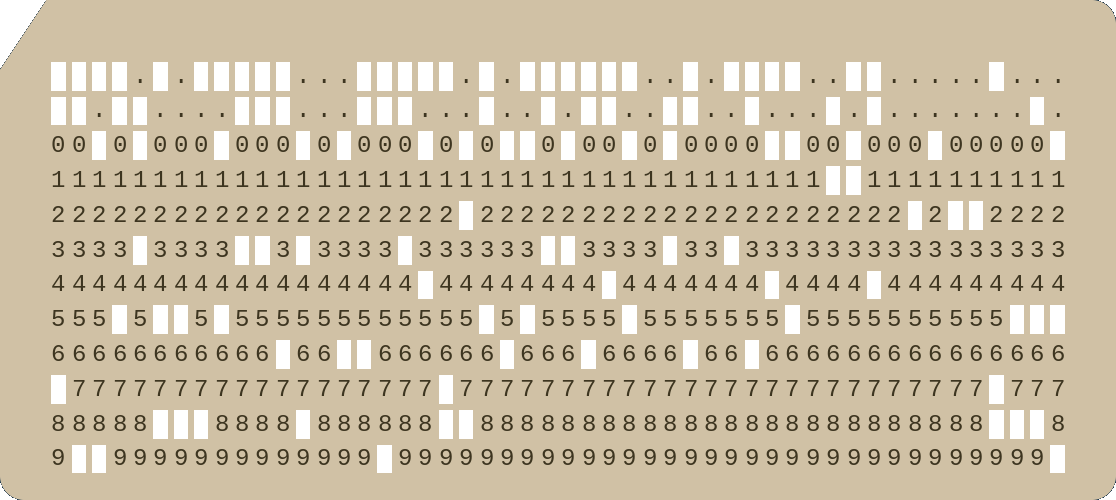The ASCII art of a punched card they want to draw is similar to an $$\mathbf{R} \times \mathbf{C}$$$matrix without the top-left cell. That means, it has $$(\mathbf{R} \cdot \mathbf{C}) - 1$$$ cells in total. Each cell is drawn in ASCII art as a period (.) surrounded by dashes (-) above and below, pipes (|) to the left and right, and plus signs (+) for each corner. Adjacent cells share the common characters in the border. Periods (.) are used to align the cells in the top row.

For example, the following is a punched card with $$\mathbf{R} = 3$$$rows and $$\mathbf{C} = 4$$$ columns:

..+-+-+-+
..|.|.|.|
+-+-+-+-+
|.|.|.|.|
+-+-+-+-+
|.|.|.|.|
+-+-+-+-+


There are more examples with other sizes in the samples below. Given the integers $$\mathbf{R}$$$and $$\mathbf{C}$$$ describing the size of a punched card, print the ASCII art drawing of it as described above.

### Input

The first line of the input gives the number of test cases, $$\mathbf{T}$$$. $$\mathbf{T}$$$ lines follow, each describing a different test case with two integers $$\mathbf{R}$$$and $$\mathbf{C}$$$: the number of rows and columns of the punched card that must be drawn.

### Output

For each test case, output one line containing Case #$$x$$$:, where $$x$$$ is the test case number (starting from 1). Then, output $$(2 \cdot \mathbf{R}) + 1$$$additional lines with the ASCII art drawing of a punched card with $$\mathbf{R}$$$ rows and $$\mathbf{C}$$$columns. ### Limits Time limit: 5 seconds. Memory limit: 1 GB. #### Test Set 1 (Visible Verdict) $$1 \le \mathbf{T} \le 81$$$.
$$2 \le \mathbf{R} \le 10$$$. $$2 \le \mathbf{C} \le 10$$$.

### Sample

Sample Input
3
3 4
2 2
2 3

Sample Output
Case #1:
..+-+-+-+
..|.|.|.|
+-+-+-+-+
|.|.|.|.|
+-+-+-+-+
|.|.|.|.|
+-+-+-+-+
Case #2:
..+-+
..|.|
+-+-+
|.|.|
+-+-+
Case #3:
..+-+-+
..|.|.|
+-+-+-+
|.|.|.|
+-+-+-+


Sample Case #1 is the one described in the problem statement. Sample Cases #2 and #3 are additional examples. Notice that the output for each case contains exactly $$\mathbf{R} \cdot \mathbf{C} + 3$$$periods. ## B. 3D Printing ### Problem You are part of the executive committee of the Database Design Day festivities. You are in charge of promotions and want to print three D's to create a logo of the contest. You can choose any color you want to print them, but all three have to be printed in the same color.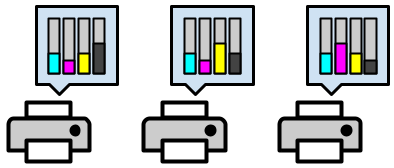You were given three printers and will use each one to print one of the D's. All printers use ink from $$4$$$ individual cartridges of different colors (cyan, magenta, yellow, and black) to form any color. For these printers, a color is uniquely defined by $$4$$$non-negative integers $$c$$$, $$m$$$, $$y$$$, and $$k$$$, which indicate the number of ink units of cyan, magenta, yellow, and black ink (respectively) needed to make the color. The total amount of ink needed to print a single D is exactly $$10^6$$$ units. For example, printing a D in pure yellow would use $$10^6$$$units of yellow ink and $$0$$$ from all others. Printing a D in the Code Jam red uses $$0$$$units of cyan ink, $$500000$$$ units of magenta ink, $$450000$$$units of yellow ink, and $$50000$$$ units of black ink.

To print a color, a printer must have at least the required amount of ink for each of its $$4$$$color cartridges. Given the number of units of ink each printer has in each cartridge, output any color, defined as $$4$$$ non-negative integers that add up to $$10^6$$$, such that all three printers have enough ink to print it. ### Input The first line of the input gives the number of test cases, $$\mathbf{T}$$$. $$\mathbf{T}$$$test cases follow. Each test case consists of $$3$$$ lines. The $$i$$$-th line of a test case contains $$4$$$ integers $$\mathbf{C_i}$$$, $$\mathbf{M_i}$$$, $$\mathbf{Y_i}$$$, and $$\mathbf{K_i}$$$, representing the number of ink units in the $$i$$$-th printer's cartridge for the colors cyan, magenta, yellow, and black, respectively. ### Output For each test case, output one line containing Case #$$x$$$: $$r$$$, where $$x$$$ is the test case number (starting from 1) and $$r$$$is IMPOSSIBLE if there is no color that can be printed by all $$3$$$ printers. Otherwise, $$r$$$must be equal to "$$c$$$ $$m$$$$$y$$$ $$k$$$" where $$c$$$, $$m$$$, $$y$$$, and $$k$$$are non-negative integers that add up to $$10^6$$$ and $$c \le \mathbf{C_i}$$$, $$m \le \mathbf{M_i}$$$, $$y \le \mathbf{Y_i}$$$, and $$k \le \mathbf{K_i}$$$, for all $$i$$$. If there are multiple solutions, you may output any one of them. (See "What if a test case has multiple correct solutions?" in the Competing section of the FAQ.) This information about multiple solutions will not be explicitly stated in the remainder of the 2022 contest. ### Limits Time limit: 5 seconds. Memory limit: 1 GB. #### Test Set 1 (Visible Verdict) $$1 \le \mathbf{T} \le 100$$$.
$$0 \le \mathbf{C_i} \le 10^6$$$, for all $$i$$$.
$$0 \le \mathbf{M_i} \le 10^6$$$, for all $$i$$$.
$$0 \le \mathbf{Y_i} \le 10^6$$$, for all $$i$$$.
$$0 \le \mathbf{K_i} \le 10^6$$$, for all $$i$$$.

### Sample

Sample Input
3
300000 200000 300000 500000
300000 200000 500000 300000
300000 500000 300000 200000
1000000 1000000 0 0
0 1000000 1000000 1000000
999999 999999 999999 999999
768763 148041 178147 984173
699508 515362 534729 714381
949704 625054 946212 951187

Sample Output
Case #1: 300000 200000 300000 200000
Case #2: IMPOSSIBLE
Case #3: 400001 100002 100003 399994


Sample Case #1 is the image provided above. The proposed color is using up all of the ink in the cyan, magenta, and yellow cartridges of the first printer and all of the ink in the black cartridge of the last printer. This means that no additional unit of ink could be used from any of the $$4$$$ink colors, so the given sample output is the only possible output for this case. In Sample Case #2, magenta is the only color that both the first and second printers have, so our only chance would be to use $$10^6$$$ units of magenta. Unfortunately, the third printer does not have quite enough, making this case impossible.

The first line of the input gives the number of test cases, $$\mathbf{T}$$$. $$\mathbf{T}$$$ test cases follow. Each test case is described in two lines. The first line of a test case contains a single integer $$\mathbf{N}$$$, the number of dice in the game. The second line contains $$\mathbf{N}$$$ integers $$\mathbf{S_1}, \mathbf{S_2}, \dots, \mathbf{S_N}$$$, each representing the number of sides of a different die. ### Output For each test case, output one line containing Case #$$x$$$: $$y$$$, where $$x$$$ is the test case number (starting from 1) and $$y$$$is the maximum number of input dice that can be put in a straight. ### Limits Memory limit: 1 GB. $$1 \le \mathbf{T} \le 100$$$.

#### Test Set 1 (Visible Verdict)

Time limit: 5 seconds.
$$1 \le \mathbf{N} \le 10$$$. $$4 \le \mathbf{S_i} \le 20$$$, for all $$i$$$. #### Test Set 2 (Visible Verdict) Time limit: 15 seconds. $$1 \le \mathbf{N} \le 10^5$$$.
$$4 \le \mathbf{S_i} \le 10^6$$$, for all $$i$$$.

### Sample

Sample Input
4
4
6 10 12 8
6
5 4 5 4 4 4
10
10 10 7 6 7 4 4 5 7 4
1
10

Sample Output
Case #1: 4
Case #2: 5
Case #3: 9
Case #4: 1


In Sample Case #1, there are multiple ways to form a straight using all $$4$$$dice. One possible way is shown in the image above. In Sample Case #2, since none of the dice can show an integer greater than $$5$$$, there is no way to have a straight with more than $$5$$$dice. There are multiple ways to form a straight with exactly $$5$$$ dice. For example, pick the integers $$4$$$and $$5$$$ for both $$d5$$$⁠'s and then integers $$1, 2,$$$ and $$3$$$for three of the $$d4$$$⁠'s to form $$1,2,3,4,5$$$. In Sample Case #3, it is possible to form the straight $$1,2,3,4,5,6,7,8,9$$$ by discarding one $$d4$$$and using the $$d4$$$⁠'s, $$d5$$$, and $$d6$$$ to get $$1$$$through $$4$$$; the $$d7$$$⁠'s to get $$5$$$ through $$7$$$; and the $$d10$$$⁠'s to get $$8$$$and $$9$$$. There is no way to form a straight of length $$10$$$, so this is the best that can be done. In Sample Case #4, we can only form a straight of length $$1$$$, but we can do so by picking any integer for the $$d10$$$we are given. ## D. Chain Reactions ### Problem Wile lives alone in the desert, so he entertains himself by building complicated machines that run on chain reactions. Each machine consists of $$\mathbf{N}$$$ modules indexed $$1, 2, \dots, \mathbf{N}$$$. Each module may point at one other module with a lower index. If not, it points at the abyss. Modules that are not pointed at by any others are called initiators. Wile can manually trigger initiators. When a module is triggered, it triggers the module it is pointing at (if any) which in turn may trigger a third module (if it points at one), and so on, until the chain would hit the abyss or an already triggered module. This is called a chain reaction. Each of the $$\mathbf{N}$$$ modules has a fun factor $$\mathbf{F_i}$$$. The fun Wile gets from a chain reaction is the largest fun factor of all modules that triggered in that chain reaction. Wile is going to trigger each initiator module once, in some order. The overall fun Wile gets from the session is the sum of the fun he gets from each chain reaction. For example, suppose Wile has $$4$$$ modules with fun factors $$\mathbf{F_1}=60, \mathbf{F_2}=20, \mathbf{F_3}=40,$$$and $$\mathbf{F_4}=50$$$ and module $$1$$$points at the abyss, modules $$2$$$ and $$3$$$at module $$1$$$, and module $$4$$$at module $$2$$$. There are two initiators ($$3$$$and $$4$$$) that Wile must trigger, in some order.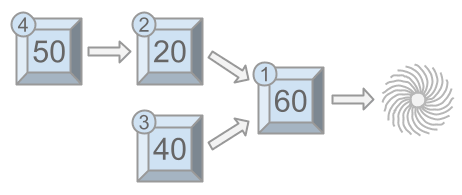As seen above, if Wile manually triggers module $$4$$$first, modules $$4$$$, $$2$$$, and $$1$$$ will get triggered in the same chain reaction, for a fun of $$\max(50, 20, 60) = 60$$$. Then, when Wile triggers module $$3$$$, module $$3$$$will get triggered alone (module $$1$$$ cannot get triggered again), for a fun of $$40$$$, and an overall fun for the session of $$60+40=100$$$.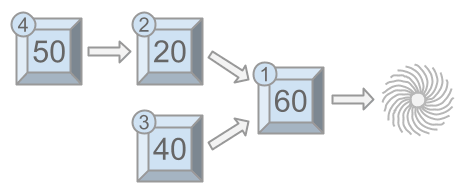### Output

For each test case, output one line containing Case #$$x$$$: $$y$$$, where $$x$$$is the test case number (starting from 1) and $$y$$$ is the maximum fun Wile can have by manually triggering the initiators in the best possible order.

### Limits

Memory limit: 1 GB.
$$1 \le \mathbf{T} \le 100$$$. $$1 \le \mathbf{F_i} \le 10^9$$$.
$$0 \le \mathbf{P_i} \le i - 1$$$, for all $$i$$$.

#### Test Set 1 (Visible Verdict)

Time limit: 5 seconds.

#### Test Set 3 (Hidden Verdict)

Time limit: 10 seconds.
$$1 \le \mathbf{N} \le 100000$$$. ### Sample Sample Input 3 4 60 20 40 50 0 1 1 2 5 3 2 1 4 5 0 1 1 1 0 8 100 100 100 90 80 100 90 100 0 1 2 1 2 3 1 3  Sample Output Case #1: 110 Case #2: 14 Case #3: 490  Sample Case #1 is the one explained in the problem statement. In Sample Case #2, there are $$4$$$ initiators (modules $$2$$$through $$5$$$), so there are $$4$$$chain reactions. Activating them in order $$3, 5, 4, 2$$$ yields chains of fun $$3, 5, 4, 2$$$for an overall fun of $$14$$$. Notice that we are summing the four highest fun numbers in the input, so there is no way to get more than that.

In Sample Case #3, an optimal activation order of the $$5$$$initiators is $$4, 5, 7, 6, 8$$$.

## E. Twisty Little Passages

### Problem

You are investigating a cave. The cave has $$\mathbf{N}$$$rooms. There are underground passages that bidirectionally connect some pairs of rooms. Each room has at least one passage connected to it. No passage goes from a room to itself, and no two rooms are connected by more than one passage. When in a room, you can identify what room you are in and see how many passages it connects to, but you cannot distinguish the passages. You want to estimate the number of passages that exist in the cave. You are allowed to do up to $$\mathbf{K}$$$ operations. An operation is either:

• be magically teleported to a room of your choice, or
• walk through a random passage connected to the room you are in, taking you to the room at the other end of that passage.

When you decide to walk through a passage, you are unable to choose which one, because they are all alike. A passage is chosen for you uniformly at random.

You begin the investigation in an arbitrary room. Estimate the number of passages between rooms in the cave with at most $$\mathbf{K}$$$operations. If $$E$$$ is your estimate and $$P$$$is the actual number of passages, your solution is considered correct for a test case if and only if $$P \cdot 2/3 \le E \le P \cdot 4/3$$$.

To pass a test set, your solution must be correct for at least 90% of the test cases in that set.

### Input and output

This is an interactive problem. You should make sure you have read the information in the Interactive Problems section of our FAQ.

Initially, your program should read a single line containing an integer, $$\mathbf{T}$$$, the number of test cases. Then, $$\mathbf{T}$$$ test cases must be processed.

For each test case, your program must first read a line containing two integers $$\mathbf{N}$$$and $$\mathbf{K}$$$: the number of rooms in the cave, and the maximum number of room operations you are allowed. Rooms are numbered between $$1$$$and $$\mathbf{N}$$$. The cave is determined at the beginning of the test case – it won't be changed while you explore it. Then, your program must process up to $$\mathbf{K} + 1$$$exchanges. The $$i$$$-th exchange starts with you reading a line containing two integers $$\mathbf{R_i}$$$and $$\mathbf{P_i}$$$, representing the number of the room you are currently in and the number of passages it connects to. Then, you must output a single line containing one of the following:

• A single uppercase W: this means you want to walk through a random passage.
• A single uppercase T and an integer $$S$$$: this means you want to teleport to room $$S$$$.
• A single uppercase E and an integer $$E$$$: this means you want to finish exploring and estimate that the cave contains $$E$$$ passages.

After an estimation operation, the judge will immediately start the next test case if there is one, regardless of the correctness of your estimation. If there is no next test case, the judge will wait for you to finish without any further output.

If the judge receives an invalidly formatted line from your program at any moment, or if your $$(\mathbf{K}+1)$$$-th exchange for a test case is not an estimation operation, the judge will print a single number $$-1$$$ and will not print any further output. If your program continues to wait for the judge after receiving a $$-1$$$, your program will time out, resulting in a Time Limit Exceeded error. Notice that it is your responsibility to have your program exit in time to receive a Wrong Answer judgment instead of a Time Limit Exceeded error. As usual, if the memory limit is exceeded, or your program gets a runtime error, you will receive the appropriate judgment. ### Limits Time limit: 120 seconds. Memory limit: 1 GB. #### Test Set 1 (Visible Verdict) $$1 \le \mathbf{T} \le 100$$$.
$$2 \le \mathbf{N} \le 10^5$$$. $$K = 8000$$$.
Each room has at least one passage connected to it.

### Testing Tool

You can use this testing tool to test locally or on our platform. To test locally, you will need to run the tool in parallel with your code; you can use our interactive runner for that. For more information, read the instructions in comments in that file, and also check out the Interactive Problems section of the FAQ.

Instructions for the testing tool are included in comments within the tool. We encourage you to add your own test cases. Please be advised that although the testing tool is intended to simulate the judging system, it is NOT the real judging system and might behave differently. If your code passes the testing tool but fails the real judge, please check the Coding section of the FAQ to make sure that you are using the same compiler as us.

Sample Interaction
Judge
Solution
1

5 3

Judge gives $$\mathbf{N}=5, \mathbf{K}=3$$$. 4 1  We start at room $$4$$$ which has $$1$$$passage. T 5  Teleport to room 5. 5 2  It has two passages. W  Walk through a passage. 4 1  We arrived at room 4 again. T 1  Teleport to room 1. 1 3  It has three passages. E 5  Guess 5 passages. (It can be shown that the actual number of passages is either 4 or 5. The two possible graphs for this test case are shown below.)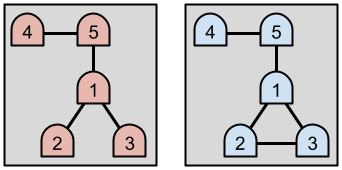## Analysis — A. Punched Cards This problem required us to print out ASCII pictures of punched cards of different sizes. Although the problem and solution are relatively straightforward, we need to know how to read from stdin and write to stdout. Examples of this can be found in the "Coding" section of the FAQ. Because the dimensions of the punched cards we need to print are so small, one option would be to store all the ASCII pictures in an array and just print the requested cards. However, we can make use of nested for-loops to generate the art for any sized punched card. We can use a loop to print out the punched card one line at a time and use another loop to print the characters in each line. The odd-numbered (1-indexed) lines alternate between (+) and (-), and the other lines alternate between (|) and (.). We can check the line number and column number $$(\text{mod} \, 2)$$$ to see which character we should print. The only exception is when both the line number and column number are $$\le 2$$$⁠. In this case, we always print a period (.). As some of you may have already discovered, for this year's qualification round, you could submit solutions in Punched Card Python. The following is a solution to Punched Cards written using Punched Card Python: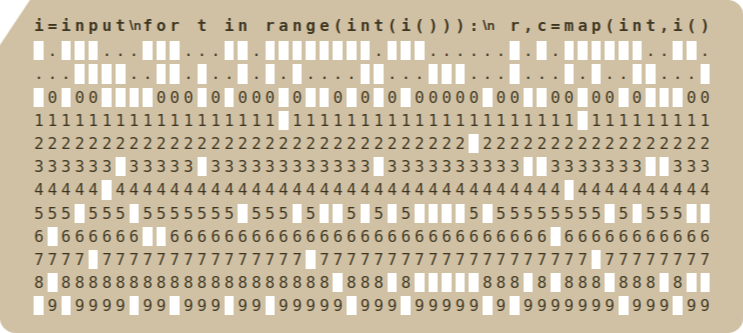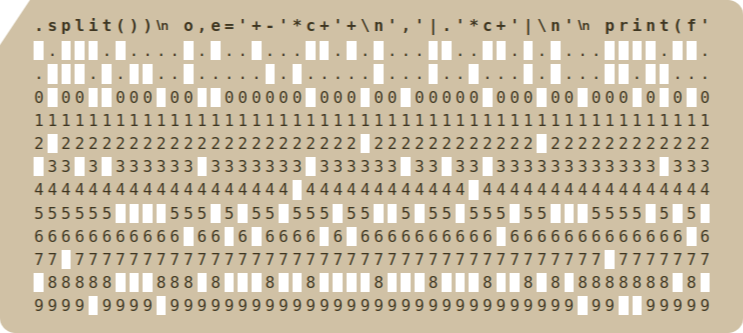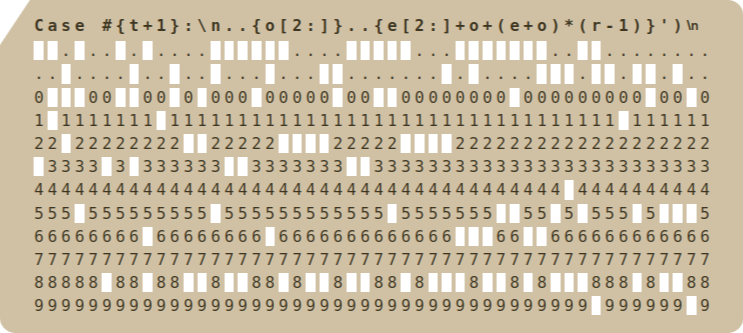Test Data info We recommend that you practice debugging solutions without looking at the test data. ## Analysis — B. 3D Printing The first thing we can notice is that if a printer has $$u$$$ units of ink left of a given color, we cannot use more than $$u$$$units of that color. Moreover, this is the only restriction imposed by that value. So, we can summarize the input by saying we cannot use more than $$C = \min(\mathbf{C_1}, \mathbf{C_2}, \mathbf{C_3})$$$ units of cyan ink, $$M = \min(\mathbf{M_1}, \mathbf{M_2}, \mathbf{M_3})$$$units of magenta ink, $$Y = \min(\mathbf{Y_1}, \mathbf{Y_2}, \mathbf{Y_3})$$$ units of yellow ink, or $$K = \min(\mathbf{K_1}, \mathbf{K_2}, \mathbf{K_3})$$$units of black ink. If $$C + M + Y + K \lt 10^6$$$ then the case is impossible and we are done. Otherwise, we may need to use lower amounts of each color. We can simply go one color at a time, lowering the amounts of ink until we make the sum exactly $$10^6$$$. Doing it one unit at a time works, but it is very slow. We can do better: in the same way as before, we can consider all the colors one at at a time. Let $$S$$$ be the sum of the current amount of ink for the $$3$$$colors not currently under consideration. If $$S \ge 10^6$$$, we can simply set the amount of the current color to $$0$$$and continue with the next one. If $$S \lt 10^6$$$ we can lower the current color to $$10^6 - S$$$and finish immediately. This works because at all times we maintain the invariant that the total amount of ink we are considering is at least $$10^6$$$ units.

Test Data
info We recommend that you practice debugging solutions without looking at the test data.

## Analysis — C. d1000000

### Test Set 1

There are multiple of ways to solve Test Set 1 of this problem. A particularly funny one is to throw the solution of an old finals problem at it (even the Test Set 1 solution of that problem works).

### Test Set 2

Test Set 2 has very big numbers, so we need insights that are specific to this problem.

Insight 1. If a straight from $$A$$$to $$B$$$ can be done, then one from $$1$$$to $$B-A+1$$$ can be done as well using the same dice in the same order, since a die showing a number $$X$$$can always be used to show number $$X-A+1$$$.

Insight 2. If a straight is done with a $$di$$$showing number $$X$$$ and a $$dj$$$showing number $$X+1$$$ with $$i \gt j$$$, we can build the same straight but using $$dj$$$ for $$X$$$and $$di$$$ for $$X+1$$$. Insight 2b. Any straight that can be done, can also be done while using the dice in non-decreasing order of number of faces. Combining insights 1 and 2b gives an algorithm: start by sorting the dice. Then, in that order, try to extend the current straight if possible. Or, in pseudo-code: maximum_straight_length(S): sort(S) length = 0 for si in S: if si > length: length += 1 return length  This algorithm requires only linear time beyond sorting the input, which means $$O(\mathbf{N} \log \mathbf{N})$$$ overall. This is fast enough to pass Test Set 2.

Test Data
info We recommend that you practice debugging solutions without looking at the test data.

## Analysis — D. Chain Reactions

### Test Set 1

In Test Set 1 there are very few modules. This means that we can try all possible orders for the manual initiators, simulate the rules as explained in the statement to get the overall fun yielded by each order, and keep the maximum result from among those. Notice that modules that are pointed at the abyss and not pointed at by any other module contribute their own fun to the total no matter the order. Therefore, we can assume they all trigger in any specific order at the beginning. This brings down the number of orders to try from $$\mathbf{N}!$$$to at most $$(\mathbf{N} / 2)!$$$, which is a lot less.

### Test Set 2

We can start by modeling the problem. We can see the input as a rooted forest where the parenting relationship models the pointed at relationship. Root nodes are modules that are pointed at the abyss.

As it is often the case for problems on trees, we can solve this one efficiently with a divide and conquer approach, and the aid of memoization/dynamic programming to keep the running time small.

Notice we can solve each tree (connected component) in the forest independently. Then, we can notice that the root of the tree is triggered by the first manual initiator. So, we can try all possibilities for the first manual initiator and eliminate the path between them and the root. That leaves a lot of separated subtrees that we can solve recursively.

Formally, let $$F(i)$$$be the fun of node $$i$$$ and $$Ftree(t)$$$be the fun value of a given subtree rooted at $$t$$$. To compute $$Ftree(t)$$$, if $$t$$$ is a single node we simply return its fun $$F(t)$$$. Otherwise, we take the maximum (over all possible x) of $$\text{fun}(x, t) + \sum_{s} Ftree(s)$$$ where $$\text{fun}(x, t)$$$is the maximum fun of all nodes in the path from $$x$$$ to $$t$$$'s root and $$x$$$ is a leaf of this subtree and the summation is over all subtrees $$s$$$whose root parent in the original tree is in the mentioned path. The domain of $$F$$$ is equal to the number of subtrees, which is equal to the number of nodes of the tree. If we memoize $$F$$$, the overall running time of computing it for all subtrees of a tree of size $$k$$$ is the domain size ($$k$$$) times the time it takes to compute it for a single item in the domain, disregarding the cost of recursive calls. This is $$O(k)$$$ because of the summation, which means an overall time complexity of $$O(k^2)$$$. The worst case is when the entire input forest is a single tree, which means the time complexity of the algorithm overall is $$O(\mathbf{N}^2)$$$.

To solve Test Set 3 we continue on our modelling from Test Set 2. We observe that the answer for $$Ftree(t)$$$can be broken down to $$\text{max}(\text{fun}(x, a), F(t)) + \sum_{s} Ftree(s)$$$ where $$a$$$is one of $$t$$$'s children and $$x$$$is a leaf node. We also observe that to maximize this, we need to choose a leaf $$x$$$ which minimizes $$\text{fun}(x, a)$$$. This way we guarantee that $$\text{fun}(x, t)$$$ benefits from having $$F(t)$$$on the path, and that all other subtrees $$s$$$ have a maximum possible value. Otherwise if we pick a path other than the minimum, this means the minimum path will be considered as one of the other subtrees which reduces $$\sum_{s} Ftree(s)$$$reducing the final answer we can get. Identifiying $$x$$$ can be done using a DFS traversal solving each tree of size $$k$$$in $$O(k)$$$. Leading to an overall complexity of $$O(N)$$$. Test Data info We recommend that you practice debugging solutions without looking at the test data. ## Analysis — E. Twisty Little Passages In general, we can't just teleport to every room, because N can be much larger than K. We could teleport to a randomly-chosen subset of the rooms, calculate the average degree (number of adjoining passages) of those rooms we've visited, and assume that this is a good estimate of the average degree of all the rooms. The number of passages is half the sum of the room degrees (since each passage connects two rooms.) When can this approach yield a poor estimate? If a small subset of the rooms have a degree much higher or much lower than the median degree, then we might not visit any of them, and our estimate would be too high or too low. Cases where most rooms have high degree and a small number of rooms have low degree are not a problem, because we are judged on relative error, and the relative error in this case is low. If the average degree is 100 but we estimate that it is 103, we would only be 3% from the answer. But if the average degree is 5 and we estimate that it is 2, we would be 60% away from the answer! So consider a troublesome case where most rooms have low degree, and a small set of rooms have high degree — few enough that we are unlikely to find one of them by teleporting randomly. If these rooms contribute a significant fraction of the total number of passages, then they must connect to a significant fraction of the total set of rooms. So we can find these with high probability by repeatedly teleporting to a random room and then walking through a random passage with the "W" command. Consider a series of rounds where we alternate between teleporting to a random room with the "T" command and walking through a random passage with the "W" command. We cannot simply extrapolate the average degree for the whole cave from the average degree of all the rooms we've seen — the rooms we reach with "W" commands are not a uniform sample. We can, however, use the average degree of just the rooms we visited with the "T" command as our estimate for all the rooms we haven't visited, and then add the known degrees to that. This is sufficient to solve the problem. Another solution is to use an alternating series of "T" and "W" commands as above, and then use a technique called importance sampling. Each visit to a room gives us a sample of the average degree, but each room does not have an equal chance of being visited in any given sample. By weighting each sample appropriately, we can compute a weighted average which serves as an unbiased estimate of the total. The weights are chosen to compensate for the non-uniform probabilities of visiting each room. When we randomly choose a room and visit it via a "T" command, each room had an equal chance of being chosen; we give this sample a weight of 1. When we visit a room via a "W" command, each room did not have an equal chance of being visited. We need to calculate weights for these samples, such that the expected weight for each room (the probability of visiting that room via a "W" command, multiplied by the expected weight we assign for such visits) is $$1/N$$$. This will give our final estimate the correct expected value.

Consider a sample where we were previously in a room $$R_1$$$with degree $$A$$$, and then we issued a "W" command and walked into a room $$R_2$$$with degree $$B$$$. The probability of us being in $$R_1$$$after the last "T" command was $$1/N$$$. The probability of following the passage to $$R_2$$$was $$1/A$$$, because $$R_1$$$had $$A$$$ passages connected to it. So the overall probability was $$1/(AN)$$$. We assign this sample the weight $$A/B$$$, so that the contribution to the expected weight for room $$R_2$$$is $$1/(BN)$$$. After we sum over all $$B$$$ways we could arrive at $$R_2$$$, we get a total expected weight of $$1/N$$$for $$R_2$$$, as required.

As an example, consider the following interaction:

T 1
1 1
W
3 2
T 2
2 1
W
3 2
T 3
3 2
W
1 1

We get samples of degrees $$1, 2, 1, 2, 2, 1$$$with weights $$1, 1/2, 1, 1/2, 1, 2$$$, and the weighted average degree is $$8/6$$\$.

## Statistics — A. Punched Cards

### Test set 1: 30667 correct solutions (93.8% solve rate)

First
semiexp aka semiexp. C++, 2:13
Maksim1744 C++, 2:37
Thien C++, 2:45
ecnerwala C++, 2:47
Shortest
Sarthack Python, 185 bytes
FibonacciPrower Python, 189 bytes
Autre Ruby, 193 bytes
Linguo Python, 197 bytes
somahn Python, 198 bytes

## Statistics — B. 3D Printing

### Test set 1: 25087 correct solutions (76.7% solve rate)

First
semiexp aka semiexp. C++, 5:24
Maksim1744 C++, 5:48
Thien C++, 5:53
ecnerwala C++, 5:58
Shortest
TheJorge Ruby, 244 bytes
cielavenir Ruby, 246 bytes
fah Python, 255 bytes
angelp57 Ruby, 270 bytes
FanFly Python, 278 bytes